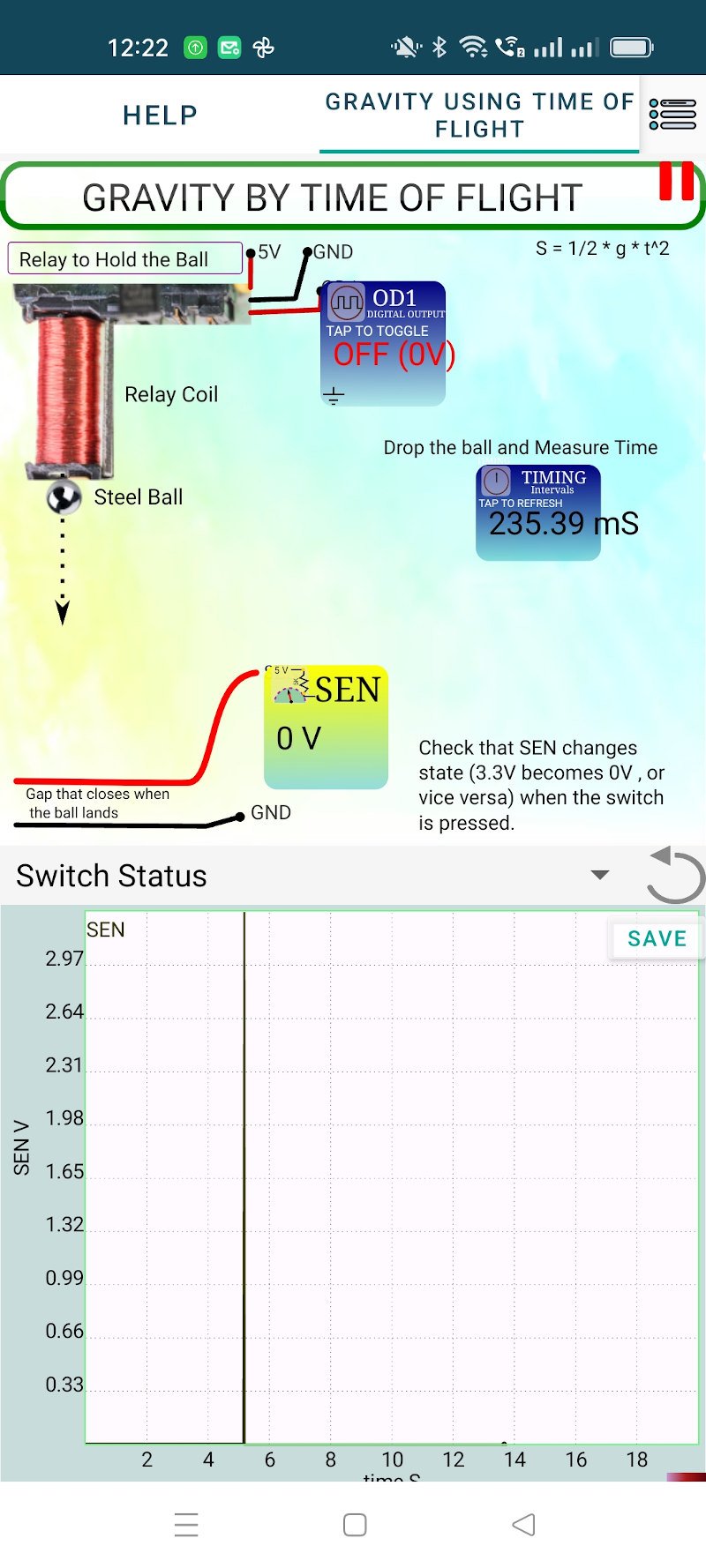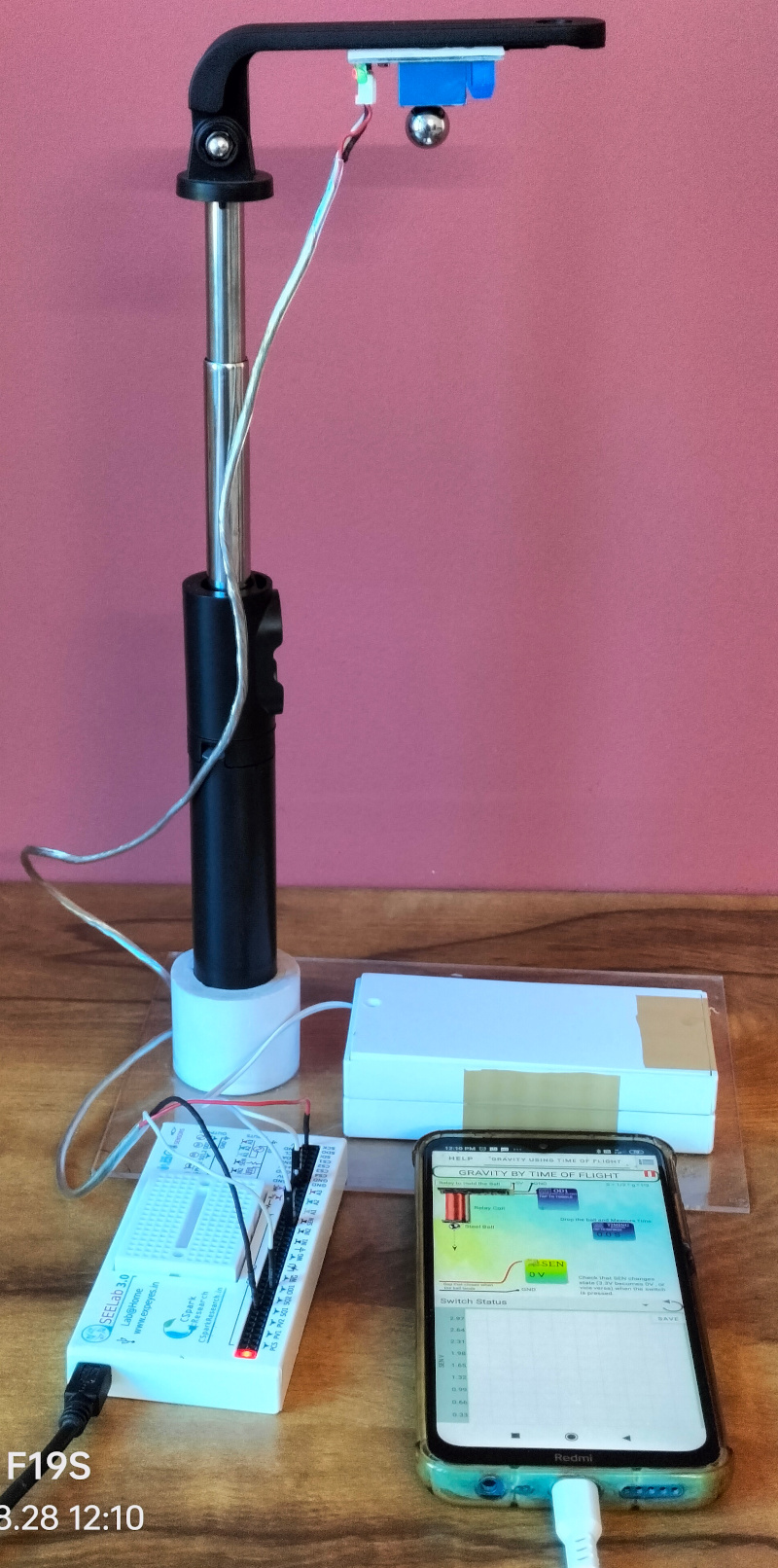# ExpEYES

## Gravity by Time of Flight

Distance travelled by a freely falling body is given by the equation $S = \frac{1}{2}gt^2 => g=\frac{2S}{t^2}$. A mild steel ball is attached to an electromagnet fixed at a height. A contact sensor is kept below. The ball is releasd under program control and the time to reach the contact sensor is measured. The experimental setup is shown below.The distance from the lowermost point to the contact sensor is 27 cm. Using the equation \$ g = \frac{2S}{t^2} = \frac{54}{0.2354^2} = 974.5 . The value of ‘g’ is very close to the actual value but there are several sources of error.

• We assume that the ball is released at the moment the voltage applied to the electromagnet is removed, but that is not true. It takes several milliseconds, due to the inductance of the coil. One can reduce the error by making the attraction just enough to hold the ball. This can be done by creating a gap between the electromagnet and the ball by placing a layer of cellotape between them.

The Python program to perform this experiment is listed below.

import eyes17.eyes
p = eyes17.eyes.open()

S = 27     # height

for k in range(5):
p.set_state(OD1 =0)			# The relay used is energized when control voltage is LOW.
input('Attach the ball and press the Enter key')
res = p.set2ftime('OD1', 'SEN')		# SEN goes LOW when the ball hits the sensor.
res -= 0.003  # 3 mS delay in releasing the ball
print(res, 2*S/res**2)